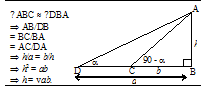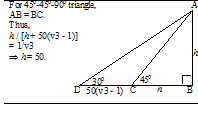# Sample Aptitude Questions of Amdocs

1. If 4x - 3y = 19 and x + 2y = 13, what are the respective values of x and y?
1. 5, 4
2. 6, 5
3. 7, 3
4. 8, 7
4x-3y=19, x+2y=13 Option 3rd satisfies all two equations
1. Due to global recession starting in January, Ram's monthly salary of Rs 8,000 was cut by 10%. The monthly expenses, which were Rs 6,000, increased at the rate of 5% per month. From which month will he have no savings if the recession lasted for a year?
1. May
2. April
3. March
4. June
 Month Salary Expenses Saving January 7200 6000+ 5% of 6000 = 6300 7200-6300-900 February 7200 6300+5% of 6300= 6615 7200-6615=585 March 7200 6615+5% of 6615= 6945.75 7200-6945.75=254.25 April 7200 6945.75+ 5% of 6945.75 = 7293.0375 Expenses are more than salary
From April onwards, he will have no savings.
2. A hemispherical bowl is 176 cm round the brim. Supposing it to be half full, how many persons may be served from it in hemispherical glasses with diameter of 4 cm at the top?
1. 1372
2. 1272
3. 1172
4. 1472
Circumference of bowl = 176 = 2 π r ; r = 28
Quantity of liquid = ½ * (2/3 * π * 283) = 3 * 283 π.
Volume of a glass is = 2/3 *π * 23 = 16π/3.
Number of glasses = (3 * 283π / (16π/3)
= 1372
3. A and B working together can complete a piece of work in 12 days. B and C working together can complete the same work in 16 days. A worked at it for 5 days and B worked at it for 7 days. C finished the remaining work in 13 days. How many days would C alone take to complete it?
1. 32
2. 24
3. 10
4. 40
1/A + 1/B = 1/12 and 1/B + 1/C = 1/16
= 5/A + 7/B + 13/C = (1/A + 1/B)5 + (1/B + 1/C )2 + 11/C = 1. Substituting from the above equations, we get
C = 24.
4. Sixteen men can complete a work in twelve days. Twenty-four children can complete the same work in eighteen days. Twelve men and eight children started working and after eight days three more children joined them. How many days will they now take to complete the remaining work?
1. 6
2. 4
3. 2
4. None of these
Let the total work = 36
A man does 36/(12 * 16) = 3/16 work per day.
A child does 36/(18 * 24) = 1/12 work per day.
12 men & 8 children do 12(3/16) + 8 (1/12) = 9/4 + 2/3
Now for 8 days, 8(9/4 + 2/3) = 70 / 3
The remaining work = 36 – (70/3) = 38/3
12 men and 11 children will complete the remaining work in x days.
x[(12 * 9/4) + (11 * 1/12)] = 38/3 * x = 4.
1. Two men A and B working together complete a piece of work which it would have taken them 30 and 40 days respectively to complete if they worked separately. If they received a payment of ` 21,000, B's share is
1. 8000
2. 12000
3. 9000
4. None of these
Let the amount of work be 120 units.
A would have done 4 units a day.
B would have done 3 units a day.
So the ratio when they work together = 4 : 3
From 21000, B' share will 3/7 Rs 21000 = 9,000.
2. If Rs 10 be allowed as true discount on a bill of Rs 110 due at the end of a certain time, then the discount allowed on the sum due at the end of double the time is
1. 22
2. 21.81
3. 20
4. 18.33
Discount of Rs.10 on Rs.110  discount = 10%.
Bill= Rs.100
After the end of double the time, Bill = 200
So, 10 % of 200 = 20.
3. A man purchased a cycle for Rs 3,000 and sold it the same day for Rs 3,600, allowing the buyer a credit of 2 years. If the rate of interest be 10% per annum, then the man has a gain of
1. 7.5%
2. 5%
3. 20%
4. None of these
The rate of interest is 10%.
For 2 years, It will become 20% of 3600 = 720.
So he gain 720 + 600 = 1320 on 3000.
So a man has a gain of 44%.
4. The angle of elevation on the top of a tower from two horizontal points at distance of 'a' and 'b' metres from the tower are 'α' and '90 -α ' respectively. The height of the tower will be (where a > b)
1. ab metres
2. √ ab metres
3. √ a/b metres
4. √ b/a metres